Comparing fractions

Chapter 7 Class 6 Fractions
Concept wise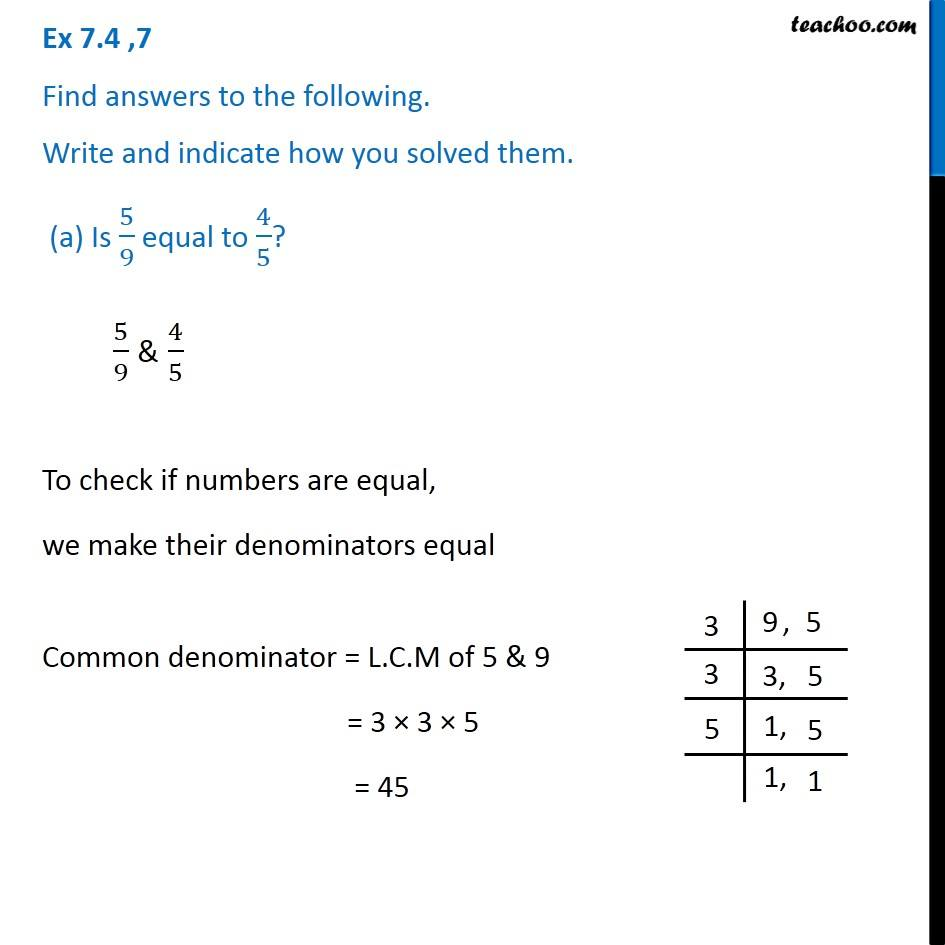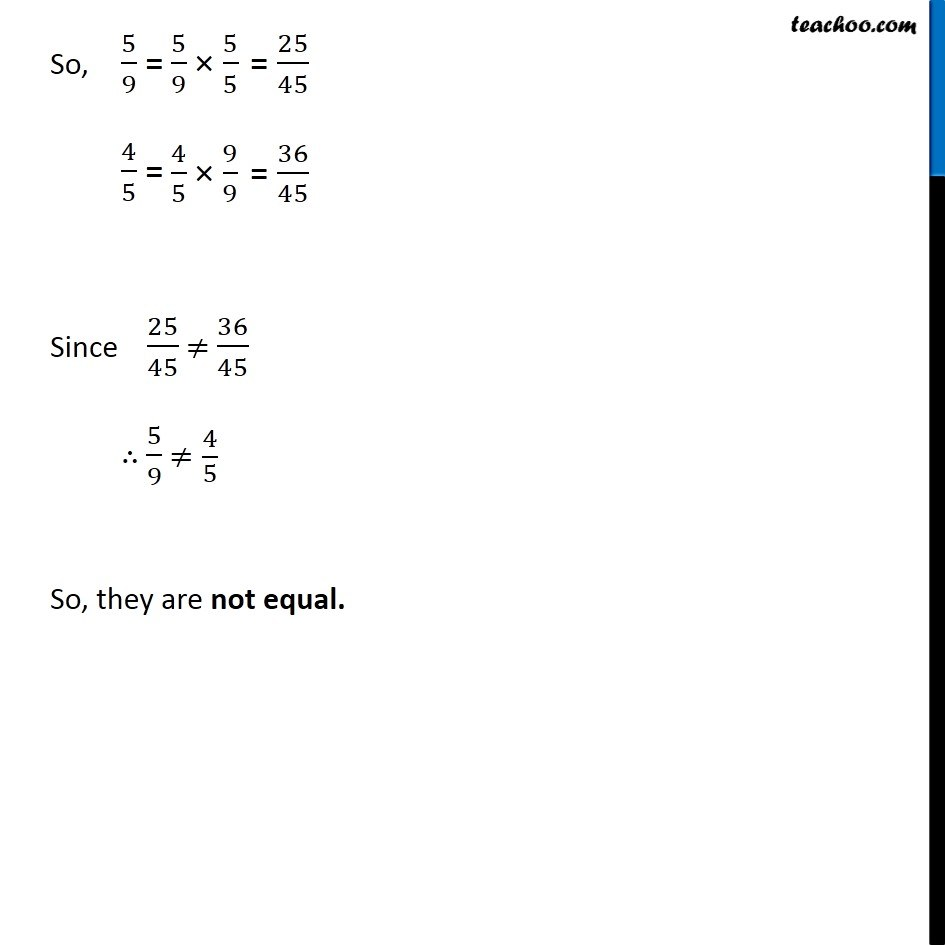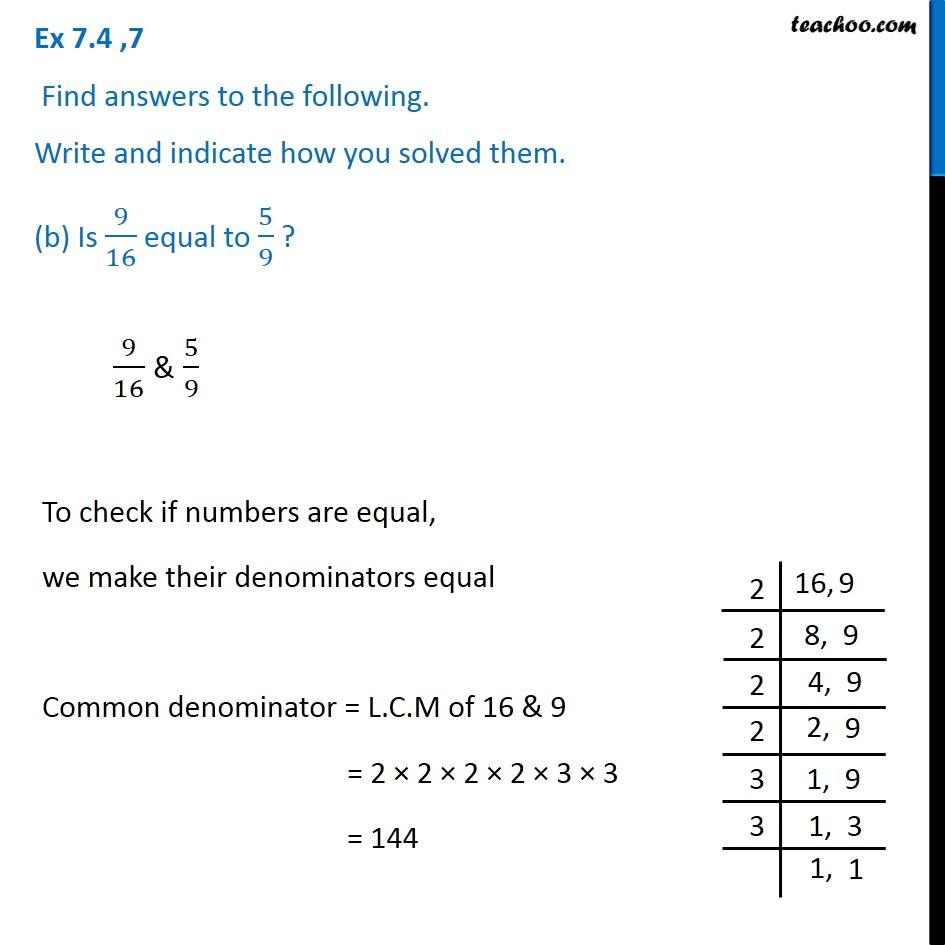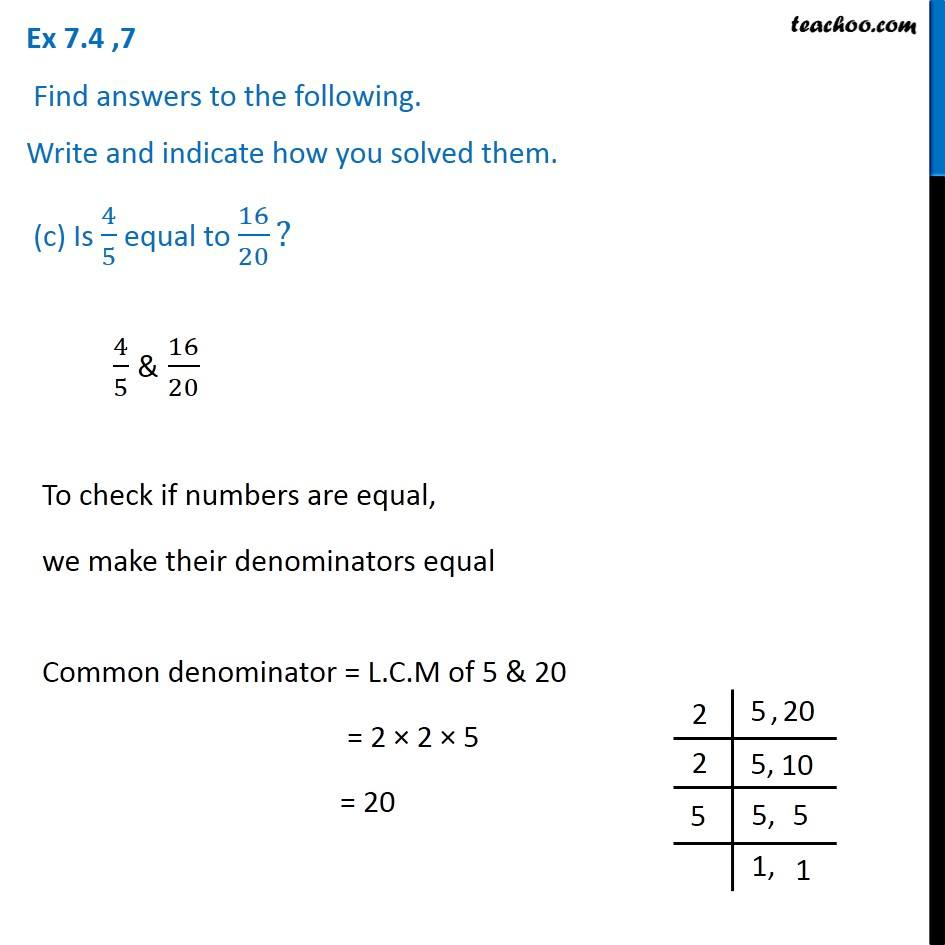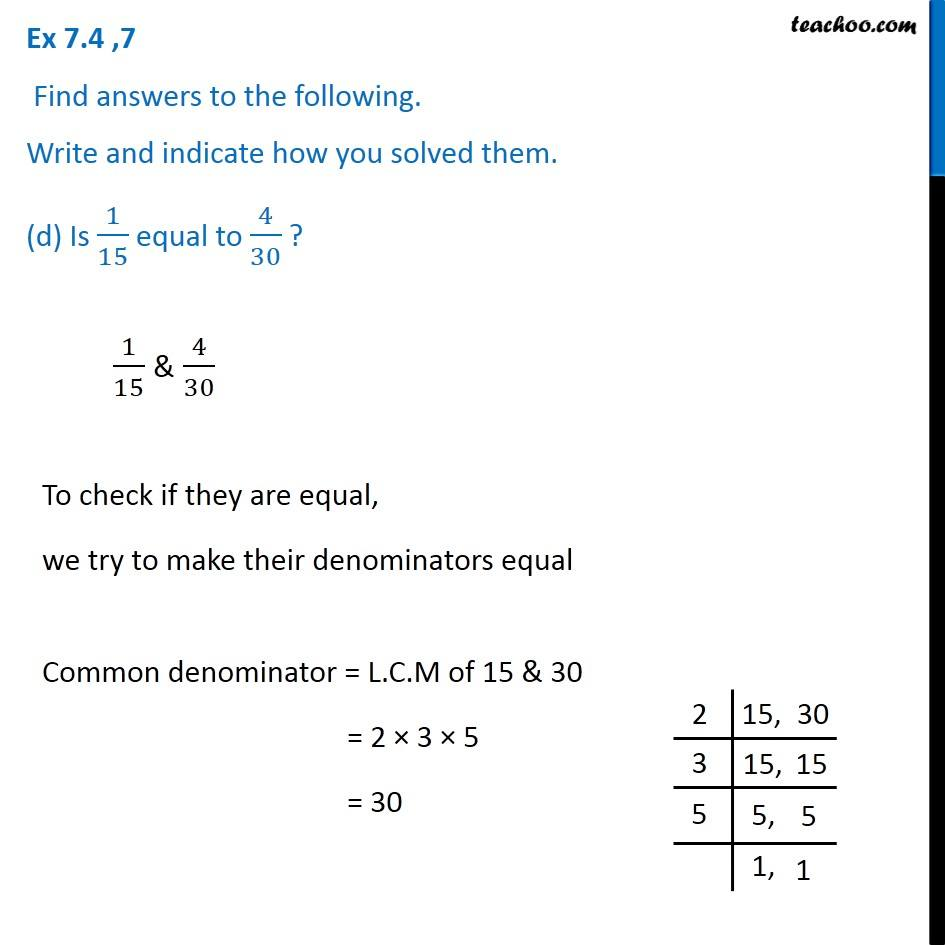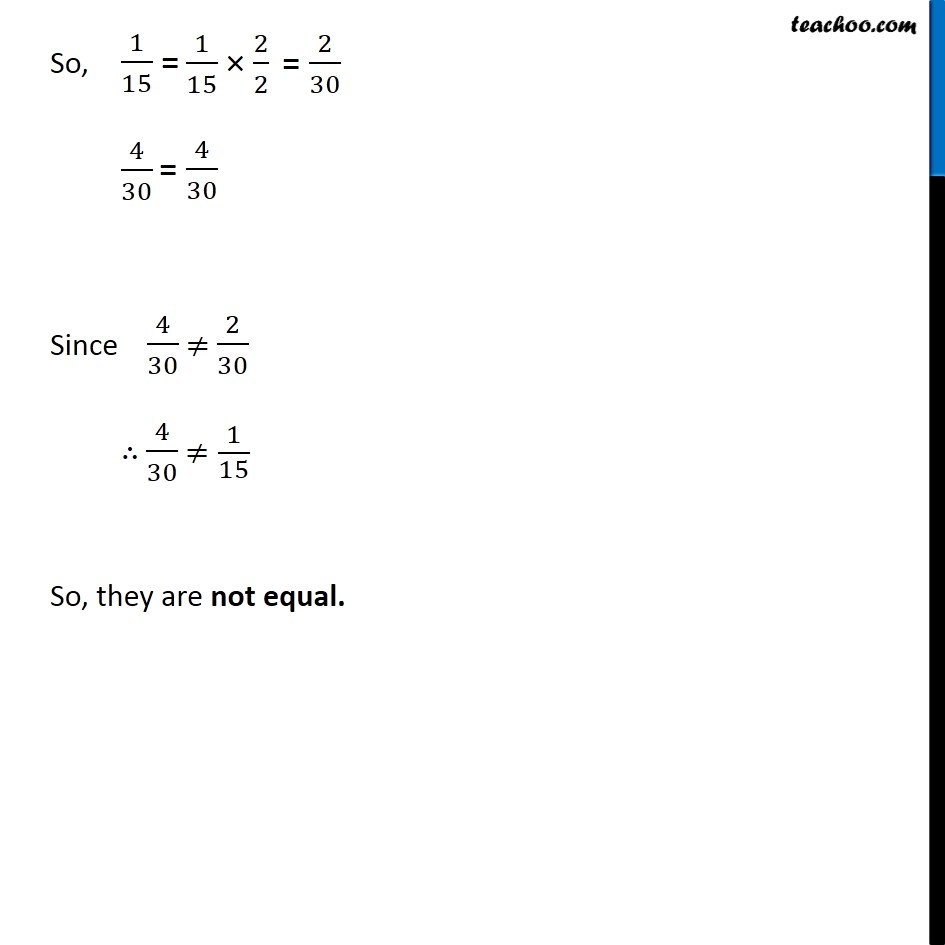Learn in your speed, with individual attention - Teachoo Maths 1-on-1 Class

### Transcript

Ex 7.4 ,7 Find answers to the following. Write and indicate how you solved them. (a) Is 5/9 equal to 4/5? 5/9 & 4/5 To check if numbers are equal, we make their denominators equal Common denominator = L.C.M of 5 & 9 = 3 × 3 × 5 = 45 So, 5/9 = 4/5 = Since 25/45 ≠ 36/45 ∴ 5/9 ≠4/5 So, they are not equal. Ex 7.4 ,7 Find answers to the following. Write and indicate how you solved them. (b) Is 9/16 equal to 5/9 ? 9/16 & 5/9 To check if numbers are equal, we make their denominators equal Common denominator = L.C.M of 16 & 9 = 2 × 2 × 2 × 2 × 3 × 3 = 144 So, 9/16 = 5/9 = Since 81/144 ≠ 80/144 ∴ 9/16 ≠5/9 So, they are not equal. Ex 7.4 ,7 Find answers to the following. Write and indicate how you solved them. (c) Is 4/5 equal to 16/20? 4/5 & 16/20 To check if numbers are equal, we make their denominators equal Common denominator = L.C.M of 5 & 20 = 2 × 2 × 5 = 20 So, 4/5 = 16/20 Since 16/20 = 16/20 ∴ 16/20 = 4/5 So, they are equal. Ex 7.4 ,7 Find answers to the following. Write and indicate how you solved them. (d) Is 1/15 equal to 4/30 ? 1/15 & 4/30 To check if they are equal, we try to make their denominators equal Common denominator = L.C.M of 15 & 30 = 2 × 3 × 5 = 30 So, 1/15 = 4/30 Since 4/30 ≠ 2/30 ∴ 4/30 ≠1/15 So, they are not equal.